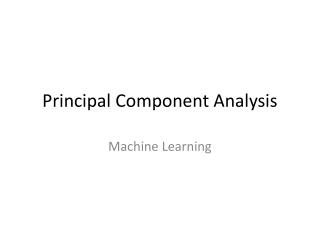DownloadDownload PresentationPrincipal Component Analysis

Principal Component Analysis

Télécharger la présentationPrincipal Component Analysis

- - - - - - - - - - - - - - - - - - - - - - - - - - - E N D - - - - - - - - - - - - - - - - - - - - - - - - - - -
Presentation Transcript

1. Principal Component Analysis Machine Learning

2. Last Time • Expectation Maximization in Graphical Models • Baum Welch

3. Now • Unsupervised Dimensionality Reduction

4. Curse of Dimensionality • In (nearly) all modeling approaches, more features (dimensions) require (a lot) more data • Typically exponential in the number of features • This is clearly seen from filling a probability table. • Topological arguments are also made. • Compare the volume of an inscribed hypersphereto a hypercube

5. Dimensionality Reduction • We’ve already seen some of this. • Regularization attempts to reduce the number of effective features used in linear and logistic regression classifiers

6. Linear Models • When we regularize, we optimize a function that ignores as many features as possible. • The “effective” number of dimensions is much smaller than D

7. Support Vector Machines • In exemplar approaches (SVM, k-nn) each data point can be considered to describe a dimension. • By selecting only those instances that maximize the margin (setting α to zero), SVMs use only a subset of available dimensions in their decision making.

8. Decision Trees weight • Decision Trees explicitly select split points based on features that improve InformationGain or Accuracy • Features that don’t contribute to the classification sufficiently are never used. <165 5M height <68 5F 1F / 1M

9. Feature Spaces • Even though a data point is described in terms of N features, this may not be the most compact representation of the feature space • Even classifiers that try to use a smaller effective feature space can suffer from the curse-of-dimensionality • If a feature has some discriminative power, the dimension may remain in the effective set.

10. 1-d data in a 2-d world

11. Dimensions of high variance

12. Identifying dimensions of variance • Assumption: directions that show high variance represent the appropriate/useful dimension to represent the feature set.

13. Aside: Normalization • Assume 2 features: • Percentile GPA • Height in cm. • Which dimension shows greater variability?

14. Aside: Normalization • Assume 2 features: • Percentile GPA • Height in cm. • Which dimension shows greater variability?

15. Aside: Normalization • Assume 2 features: • Percentile GPA • Height in m. • Which dimension shows greater variability?

16. Principal Component Analysis • Principal Component Analysis (PCA) identifies the dimensions of greatest variance of a set of data.

17. Eigenvectors • Eigenvectors are orthogonal vectors that define a space, the eigenspace. • Any data point can be described as a linear combination of eigenvectors. • Eigenvectors of a square matrix have the following property. • The associated lambda is the eigenvalue.

18. PCA • Write each data point in this new space • To do the dimensionality reduction, keep C < D dimensions. • Each data point is now represented as a vector of c’s.

19. Identifying Eigenvectors • PCA is easy once we have eigenvectors and the mean. • Identifying the mean is easy. • Eigenvectors of the covariance matrix, represent a set of direction of variance. • Eigenvalues represent the degree of the variance.

20. Eigenvectors of the Covariance Matrix • Eigenvectors are orthonormal • In the eigenspace, the Gaussian is diagonal – zero covariance. • All eigen values are non-negative. • Eigenvalues are sorted. • Larger eigenvalues, higher variance

21. Dimensionality reduction with PCA • To convert from an original data point to PCA • To reconstruct a point

22. Eigenfaces Encoded then Decoded. Efficiency can be evaluatedwith Absolute or Squared error

23. Some other (unsupervised) dimensionality reduction techniques • Kernel PCA • Distance Preserving Dimension Reduction • Maximum Variance Unfolding • Multi Dimensional Scaling (MDS) • Isomap

24. Next Time • Model Adaptation and Semi-supervised Techniques • Work on your projects.